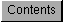```
by Carolyn Scheppner

The pixel aspect ratio describes the ratio of the width (x Aspect) to
height (y Aspect) of the pixels in a Screen or ViewPort.  In order to
create a truly What-You-See-Is-What-You-Get graphics display on the Amiga,
you need to find the pixel aspect ratio of the display mode you are using.
With the proper aspect ratio, an application can correctly display and
store ILBM files, can rotate objects properly, and can calculate the
proper dimensions to draw true circles and squares so they appear the same
on the Amiga display as they would on some other output device, like a
laser printer.

Under 1.3 and the original Amiga chip set, relatively few display modes
were available.  Under pre-2.0 versions of the OS, applications can use
hard-coded values for the X/Y pixel aspect ratio.  The aspect ratios for
the display modes available to the 1.3 system are ( xaspect / yaspect ):

NTSC Lores      44/52
PAL Lores       44/44

Halve the X aspect for Hires modes.
Halve the Y aspect for interlaced modes.

These aspect values are more accurate than the values in the original IFF
document.

On PAL displays, the pixels of Lores screens and Hires Interlace screens
are square, as they have an aspect ratio of 44/44 and 22/22, respectively.
To draw a square 100 pixels wide in one of these PAL modes, you could
simply draw a square that is 100 pixels x 100 pixels.  On a PAL Lores
Interlace display, the Y resolution is doubled, making each pixel half as
tall, so you would have to draw a rectangle that was 100 pixels x 200
pixels to get the same size square.

On an NTSC display, pixels are not square.  Pixels on a Lores NTSC screen
have an aspect ratio of 44/52 (or 11/13).  This means that each pixel is
slightly narrower than it is high.

To draw a true square that is 100 pixels wide in an arbitrary display
mode, it is necessary to calculate the correct height for the square based
on the pixel aspect ratio.

width / yAspect = height / xAspect

(in words, width is to yAspect as height is to xAspect)

If the X/Y aspect is 44/52 (Lores NTSC), the calculation would be:

100 / 52 = height / 44

height = (100 * 44) / 52 = 4400 / 52    /* solve for height */

Because this example uses only integer math, the ratio must be rounded to
the nearest integer.  A fraction a/b (where a and b are integers) rounded
to the nearest integer approximately equals:

( a + ( b / 2 ) )  /  b

apply this to the ratio above:

height = ( 4400 + (52>>1) ) / 52      /* with rounding */
height = 4426/52 = 85.115384...       /* Approximate */
height = 85                           /* truncated */

Therefore, to be square on a 44/52 aspect screen, a 100 pixel wide square
would have to be 85 pixels tall.

Under 2.0 and the ECS chip set, the Amiga display is more dynamic.  It has
many new display modes, each of which has its own distinct pixel aspect
ratio.  For this reason, it is not practical nor desirable to hard code
the aspect ratios for the modes that you know about (except when running
under 1.3).  When running under 2.0, the pixel aspect should be determined
by querying the display database (see the article ``An Introduction to V36
Screens and Windows'' from the September /October 1990 issue of Amiga Mail
for more information on how to query the display database).  A valid
DisplayInfo structure contains the X and Y aspect in the Resolution.x and
Resolution.y fields.  When writing an ILBM under 2.0, use these pixel
aspects from the display database for the BMHD chunk's xAspect and yAspect
values.

The following example getaspect.c demonstrates how to determine the X and
Y aspects under both release 2.0 and 1.3.

```

`[Back to Amiga Developer Docs]`